# T 13. Finding 6 for a givene using a graph Let f(x) = x + 3 andnote that lim f(x)3. For each value of e, use a graphing utility1x0to find all values of 6> 0 such that f(x) - 3< 8 whenever0x-0< 8. Sketch graphs illustrating your work.b. e 0.5а. Е — 1

Question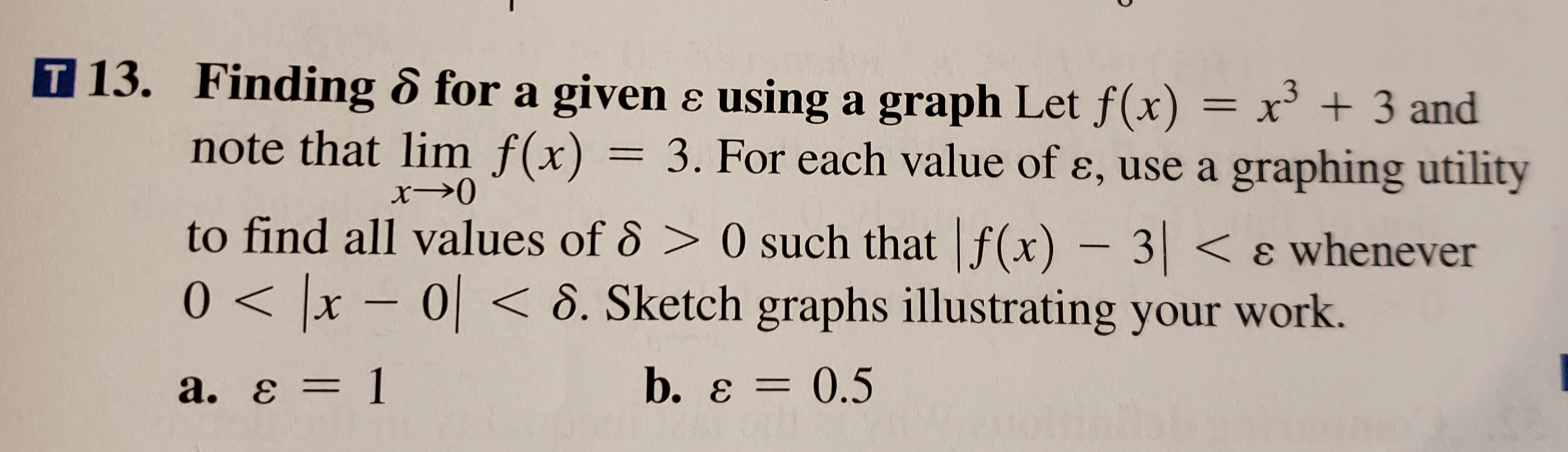help_outlineImage TranscriptioncloseT 13. Finding 6 for a givene using a graph Let f(x) = x + 3 and note that lim f(x) 3. For each value of e, use a graphing utility 1 x0 to find all values of 6> 0 such that f(x) - 3< 8 whenever 0x-0< 8. Sketch graphs illustrating your work. b. e 0.5 а. Е — 1 fullscreen
check_circleExpert Solution
Step 1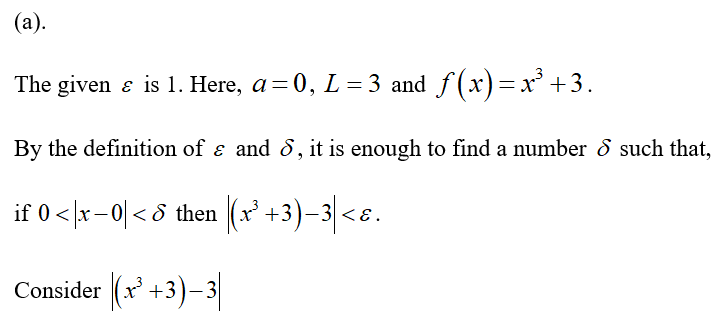Step 2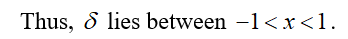Step 3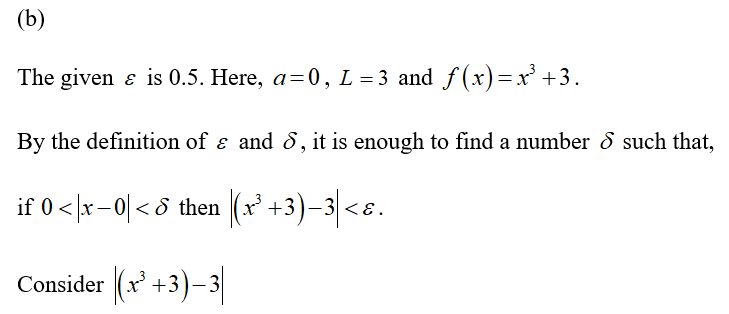...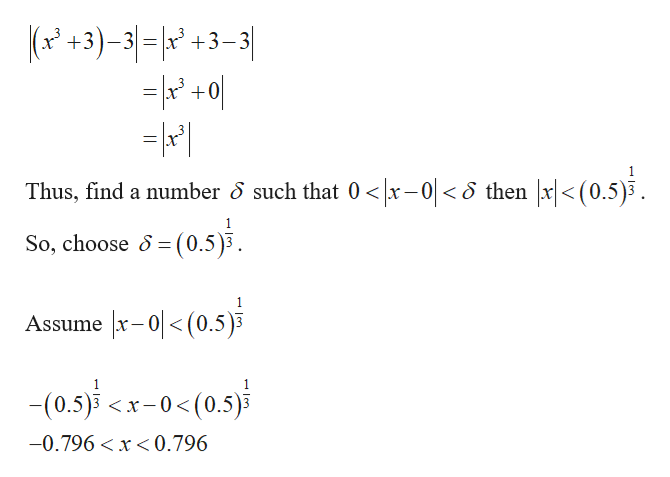help_outlineImage TranscriptioncloseP+3)-31= 12 #3 -3| x +0 Thus, find a number S such that 0 x-01< then x|<(0.5)3 So, choose (0.5)3. Assume x-<(0.5)3 -(0.5) <-0< (0.5) -0.796 < x<0.796 fullscreen

### Want to see the full answer?

See Solution

#### Want to see this answer and more?

Solutions are written by subject experts who are available 24/7. Questions are typically answered within 1 hour*

See Solution
*Response times may vary by subject and question
Tagged in

### Continuity